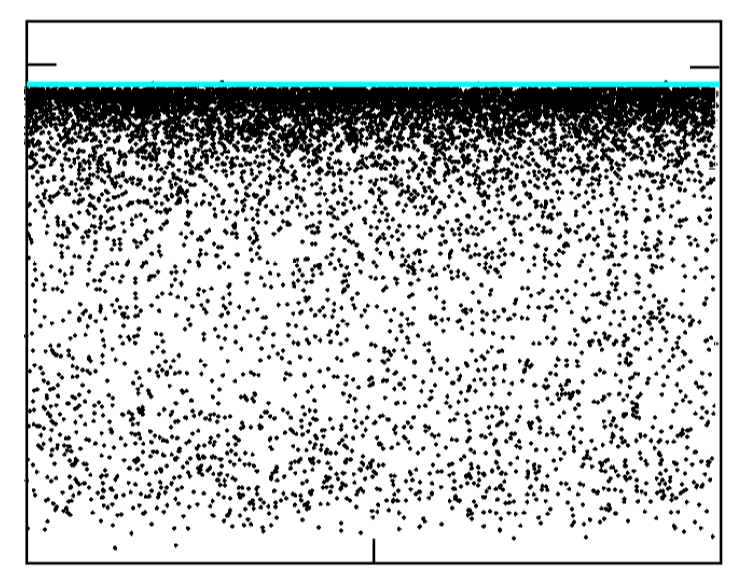# Eigenvalue estimates for operator perturbations

I edited the question to a general mathematical question, since I found the answer in Carlo Beenakker's reference and think that my initial question was mathematically misleading.

What was behind all this was: If we take $$W_N$$ being trace free skew-symmetric matrices of rank $$N$$ on an $$N$$-dimensional space. And we perturb each of them with a positive operator $$A_N$$ such that all of the $$A_N$$ have the same trace. Is it true that as $$N \rightarrow \infty$$ the real part of some eigenvalue will tend to zero (resp. be zero)?

The answer is yes by the following identity $$\operatorname{tr}(A_N)=\operatorname{Re}\operatorname{tr}(W_N+A_N) = \sum_{\lambda \in \sigma(W_N+A_N)} \operatorname{Re}(\lambda).$$

Since the left-hand side is finite, the right-hand side has to converge.

• What is your definition of "dissipative system"? If it's simply that the spectrum has negative real part I don't see why that should be true. There are purely dissipative systems for which the spectrum is and stays entirely real
– lcv
Feb 23 '19 at 21:36
• yes, that's true and yes that's my definition. However, in this particular case, there is a special dynamics that is mixing (the Laplacian connects all components of the system). Therefore, it is believable that it holds in this case. Please have a look at Carlo Beenakker's answer below and also the comments. Feb 23 '19 at 21:38
• See Carlo Beenakker's comment about $\delta N/ N$ being finite. But this is an additional assumption. I.e. if you perturb a skewhermitian with a negative operator you may (or not) end up with some imaginary eigenvalues if the perturbation is suitably small or vanishing.
– lcv
Feb 23 '19 at 21:43
• Sorry, I don't understand. $\delta N=1$ in my case. So $\delta N/N$ goes to zero. So according to him some eigenvalues should approach the imaginary axis and that is what I would like to show. I agree that this might not hold for general perturbations of skew-symmetric matrices, but the system I consider is somewhat special.-But I feel I have not understood your commet fully. Feb 23 '19 at 21:49

This problem of "non-Hermitian" quantum mechanics has been studied in the context of random-matrix theory (RMT), see for example the review Random matrix approaches to open quantum systems. The particular case pointed to in the OP is when the Hermitian matrix $${\cal H}=iW_{H}$$ of high rank $$N$$ is perturbed by a positive-definite matrix $$-i\pi WW^{t}$$ of low rank $$\delta N\ll N$$. The eigenvalues $$E_n-i\Gamma_n$$ of the effective Hamiltonian $${\cal H}_{\text{eff}}={\cal H}-i\pi WW^t$$ describe resonant scattering from a heavy atom or quantum dot (with $$E_n$$ the center of the resonance and $$\Gamma_n$$ its width). The special case in the OP where $${\cal H}$$ is block-off-diagonal is referred to as the case of "chiral symmetry" in the context of random-matrix theory. (The appropriate ensemble for real $${\cal H}$$ is the socalled "chiral orthogonal ensemble".)

Now the question in the OP is the distribution of the $$\Gamma_n$$'s in the limit $$N\rightarrow\infty$$ at fixed $$\delta N$$. The "universal" result of random-matrix theory (see section IV.A in the cited review) is that for $$\delta N\ll N$$ all $$\Gamma_n$$'s are greater than a minimal value $$\Gamma_{\text{min}}\simeq\delta N/N$$ and they accumulate near that value.
Note that the OP refers to $$i$$ times the eigenvalues of $${\cal H}$$, so this approach to the real axis corresponds to an approach to the imaginary axis in the OP.

Hence my answer to the final question of the OP, "does the spectrum approach the imaginary axis in the large-$$N$$ limit" is yes, it does, if the non-Hermitian perturbation has a rank $$\delta N$$ that remains small compared to the rank of the Hermitian part. There remains, however, for any finite $$N$$ a gap of order $$\delta N/N$$ that separates the "dissipative dynamics" from the unitary quantum mechanical evolution.

I show a plot that illustrates the clustering of the eigenvalues $$E_n-i\Gamma_n$$ of $${\cal H}_{\text{eff}}$$ near the $$\Gamma=0$$ axis, with a sharp threshold (blue horizontal line) and a gap.
In the plot $$N=500$$ and $$\delta N=50$$. There is no chiral symmetry, but that would only introduce a $$\pm E_n$$ symmetry, it would not affect the gap in the $$\Gamma$$'s.source: arXiv:1405.6896

• First, I would like to thank you for your deep insights into my question. It gives I think a lot of intuition about why this should hold. Can I however still ask you though whether the result you are referring to is rigorous?-PS: I really think what you write is very interesting for me. Feb 23 '19 at 21:26
• it is rigorous in the context of random-matrix theory, so for the gaussian ensembles; like all the RMT results, it is believed to hold for a much wider class of random matrices. Feb 23 '19 at 21:29
• ah, but so it is not necessarily clear for my deterministic sequence above, right? Feb 23 '19 at 21:31
• not necessarily so, but keep in mind that RMT was developed for deterministic systems, such as large molecules. The randomness is introduced to make the problem tractable, RMT is believed to hold for deterministic systems if they are sufficiently complex ("chaotic dynamics" is the term). It has really a remarkably wide range of applicability, it is in fact the other way around: you have to work really hard to see behavior that deviates from the universal RMT results. Feb 23 '19 at 21:34